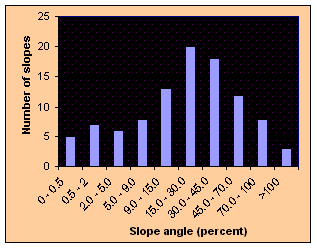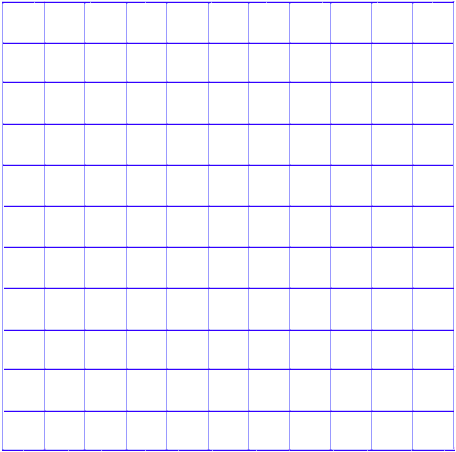# Measuring Slope Steepness

The easiest way to determine the percent slope of an area is to measure the change in height (elevation over a measured distance), then calculate the percentage of slope. Use the following formula to determine slope:

Rise ÷ Run = Slope %

OR

(Change in elevation ÷ measured distance = slope %)

e.g. 30 metres ÷ 100 metres = .3 or 30%

Calculating a slope steepness index for a map area

Stages:

• Calculate map area in km²

• Place 10 X 10 grid overlay over map

• Calculate % slope for each grid square

• Present results as an area average or median slope for the area (see chart example below)Slope Angle Index ChartTransparent grid overlay (11 X 11)

Slope (%) Approximate degrees Terminology
0 - 0.5 0 Level
0.5 - 2 0.3 - 1.1 Nearly level
2 - 5 1.1 - 3 Very gentle slope
5 - 9 3 - 5 Gentle slope
9 - 15 5 - 8.5 Moderate slope
15 - 30 8.5 - 16.5 Strong slope
30 - 45 16.5 - 24 Very strong slope
45 - 70 24 - 35 Extreme slope
70 - 100 35 - 45 Steep slope
> 100 > 45 Very steep slope

Standard slope descriptors

Slope Profile Maker using Slope Angles